# Underground Mathematics

•### Mapping diagrams with adjustable output centre

Activity

Underground Mathematics

•### Mapping diagrams

Activity

Underground Mathematics

•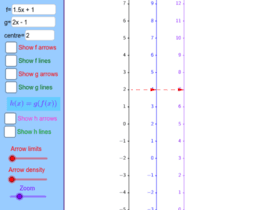### Mapping diagrams - composite functions

Activity

Underground Mathematics

•### A puzzling pentagon 2

Activity

Underground Mathematics

•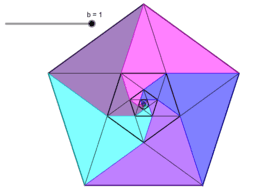### A puzzling pentagon

Activity

Underground Mathematics

•### How NOT to solve a cubic (2)

Activity

Underground Mathematics

•### How NOT to solve a cubic

Activity

Underground Mathematics

•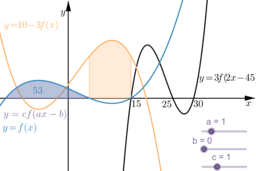### Slippery areas

Activity

Underground Mathematics

•### Happy families

Activity

Underground Mathematics

•### Make it stop problem 2

Activity

Underground Mathematics

•### Make it stop problem 1

Activity

Underground Mathematics

•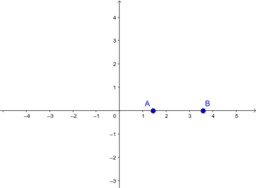Book

Underground Mathematics

•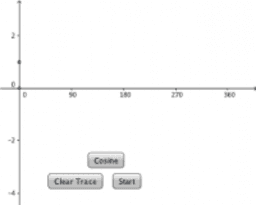### Trace the Cosine Function

Activity

Underground Mathematics

•### Trace the Sine Function

Activity

Underground Mathematics

•Activity

Underground Mathematics

•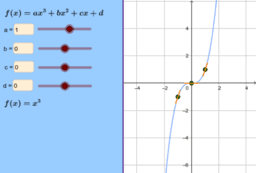Activity

Underground Mathematics

•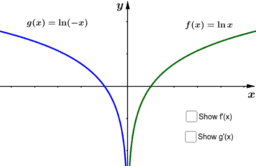### Two for one

Activity

Underground Mathematics

•Activity

Underground Mathematics

•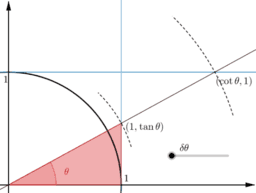### Similar derivatives

Activity

Underground Mathematics

•### sine stretching - general

Activity

Underground Mathematics

•### Sine stretching

Activity

Underground Mathematics

•### Sine stretching transforming 2

Activity

Underground Mathematics

•### Area under \$y=1/x\$

Activity

Underground Mathematics

•### Stretching the y=1/x graph (part 2)

Activity

Underground Mathematics